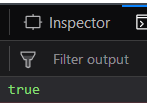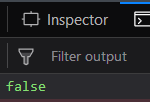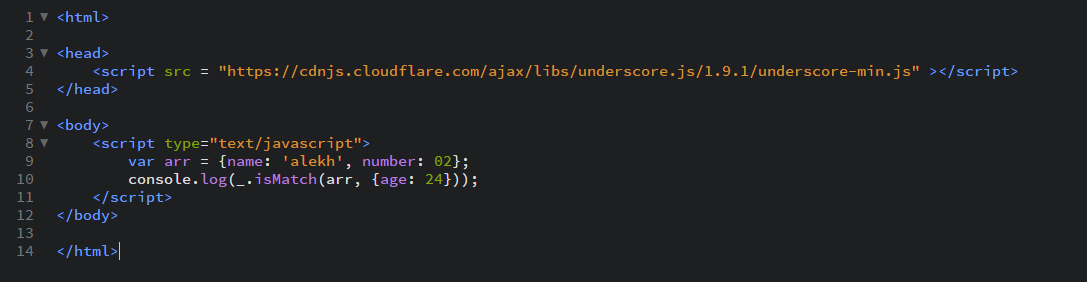Related Articles
Underscore.js | _.isMatch()
• Last Updated : 27 Dec, 2018

_.isMatch() function:

• It is used to find out if the property given in argument is present in the passed array or not.
• Also, the property’s value should be same in order to match.
• It is used in cases where we want to find whether the array satisfies a specific condition or not.

Syntax:

`_.isMatch(object, properties)`

Parameters:
It takes two arguments:

• The object/array
• The property with it’s value

Return value:
It returns true if the property along with it’s value matches the array passed otherwise returns false.

Examples:

1. Passing a number property to the _.isMatch() function:
The _.isMatch() function takes property passed in the second argument and then tries to find that property in the array passed. If the property exists in the array definition it checks and matches it’s value both in the array definition and in the second parameter. If it matches then it returns true otherwise it returns false. If the property is not mentioned in the array definition then it will simply return false.

 `` `<``html``> ` `  `  `<``head``> ` `    ``<``script` `src` `=  ` `    ``"https://cdnjs.cloudflare.com/ajax/libs/underscore.js/1.9.1/underscore-min.js"``> ` `    `` ` ` ` `  `  `<``body``> ` `    ``<``script` `type``=``"text/javascript"``> ` `        ``var arr = {name: 'alekh', number: 02}; ` `        ``console.log(_.isMatch(arr, {number: 2})); ` `    `` ` ` ` `  `  ` `

Output:2. Passing a character property to the _.isMatch() function:
It will work in the same way as the _.isMatch() function worked for the number property. Like here it will compare the strings given in the property. Here first it will check for the ‘name’ property and then it will match the name mentioned in the second parameter, i.e., ‘alekh’ to the name property in the array definition which is also ‘alekh’. Hence, the output will be true.

 `` `<``html``> ` `  `  `<``head``> ` `    ``<``script` `src` `=  ` `    ``"https://cdnjs.cloudflare.com/ajax/libs/underscore.js/1.9.1/underscore-min.js"``> ` `     `` ` ` ` `  `  `<``body``> ` `    ``<``script` `type``=``"text/javascript"``> ` `        ``var arr = {name: 'alekh', number: 02}; ` `        ``console.log(_.isMatch(arr, {name: 'alekh'})); ` `    `` ` ` ` `  `  ` `

Output:3. Passing an empty array to the _.isMatch() function:
The _.isMatch() function will see that no property is passed in the second parameter and hence will not check ahead and will simply return a true. It does not need to worry about the other properties mentioned in the array definition.

 `<``html``> ` `  `  `<``head``> ` `    ``<``script` `src` `=  ` `    ``"https://cdnjs.cloudflare.com/ajax/libs/underscore.js/1.9.1/underscore-min.js"``> ` `    `` ` ` ` `  `  `<``body``> ` `    ``<``script` `type``=``"text/javascript"``> ` `        ``var arr = {}; ` `        ``console.log(_.isMatch(arr, {})); ` `    `` ` ` ` `  `  ` `

Output:4. Passing a property which is not mentioned in the array definition to the _.isMatch() function:
If we pass the second parameter which is not mentioned in the array definition then the output will be false. This is because, the _.isMatch() function will not have any property in the definition to match from and hence the output will be false finally.

 `` `<``html``> ` `  `  `<``head``> ` `    ``<``script` `src` `=  ` `    ``"https://cdnjs.cloudflare.com/ajax/libs/underscore.js/1.9.1/underscore-min.js"``> ` `    `` ` ` ` `  `  `<``body``> ` `    ``<``script` `type``=``"text/javascript"``> ` `        ``var arr = {name: 'alekh', number: 02}; ` `        ``console.log(_.isMatch(arr, {age: 24})); ` `    `` ` ` ` `  `  ` `

Output:5. `

NOTE:
These commands will not work in Google console or in firefox as for these additional files need to be added which they didn’t have added.

 `` `<``script` `type``=``"text/javascript"` `src` `= ` `"https://cdnjs.cloudflare.com/ajax/libs/underscore.js/1.9.1/underscore-min.js"``> ` ` `

An example is shown below:My Personal Notes arrow_drop_up
Recommended Articles
Page :